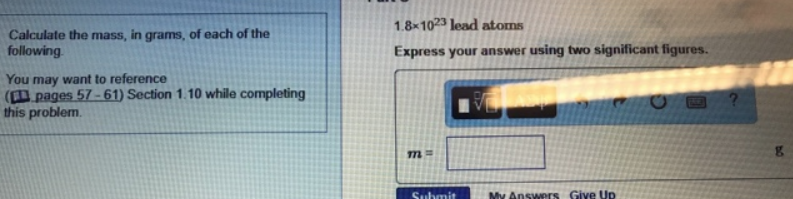# Problem: Calculate the mass, in grams of each of the following1.8x1023 lead atoms

###### FREE Expert Solution
86% (123 ratings)###### Problem Details

Calculate the mass, in grams of each of the following